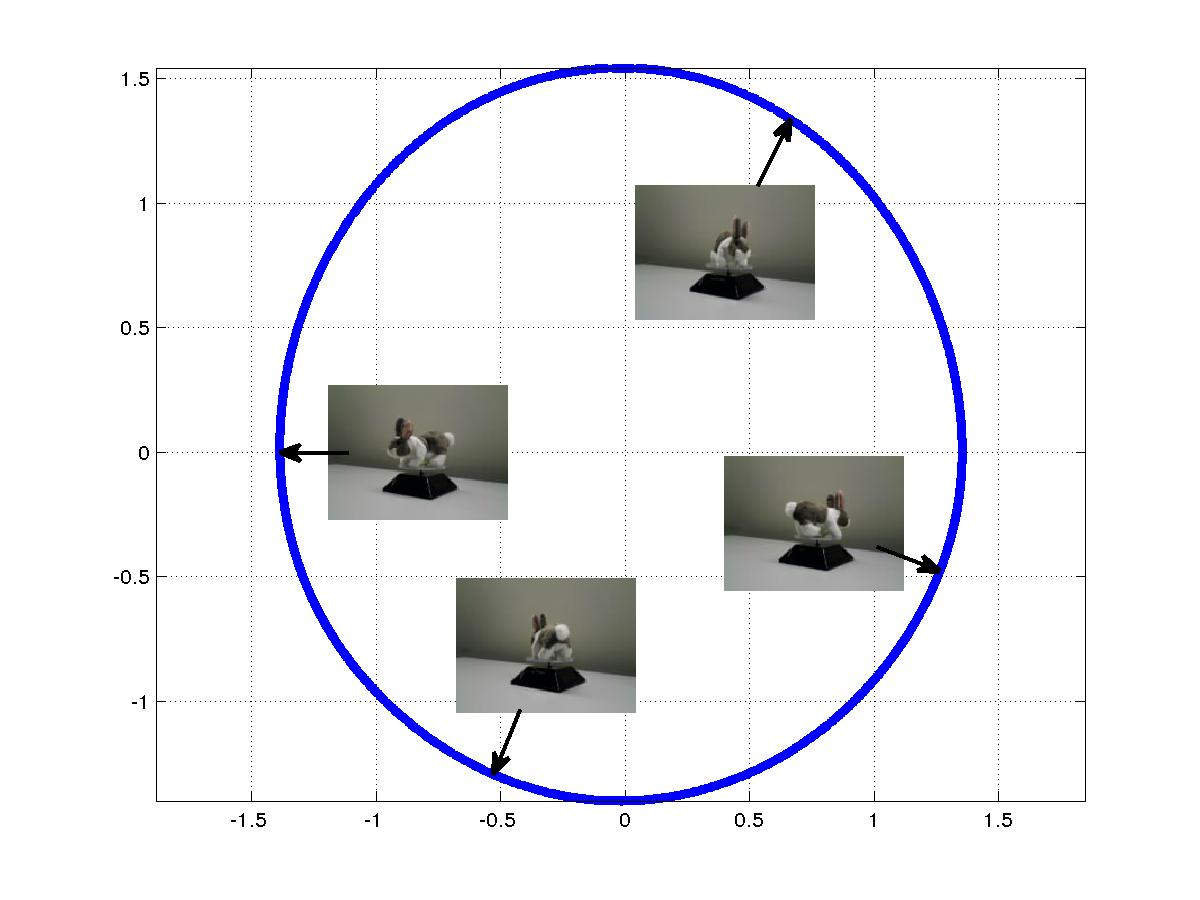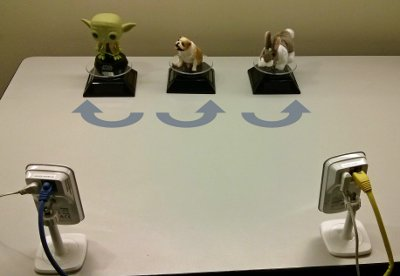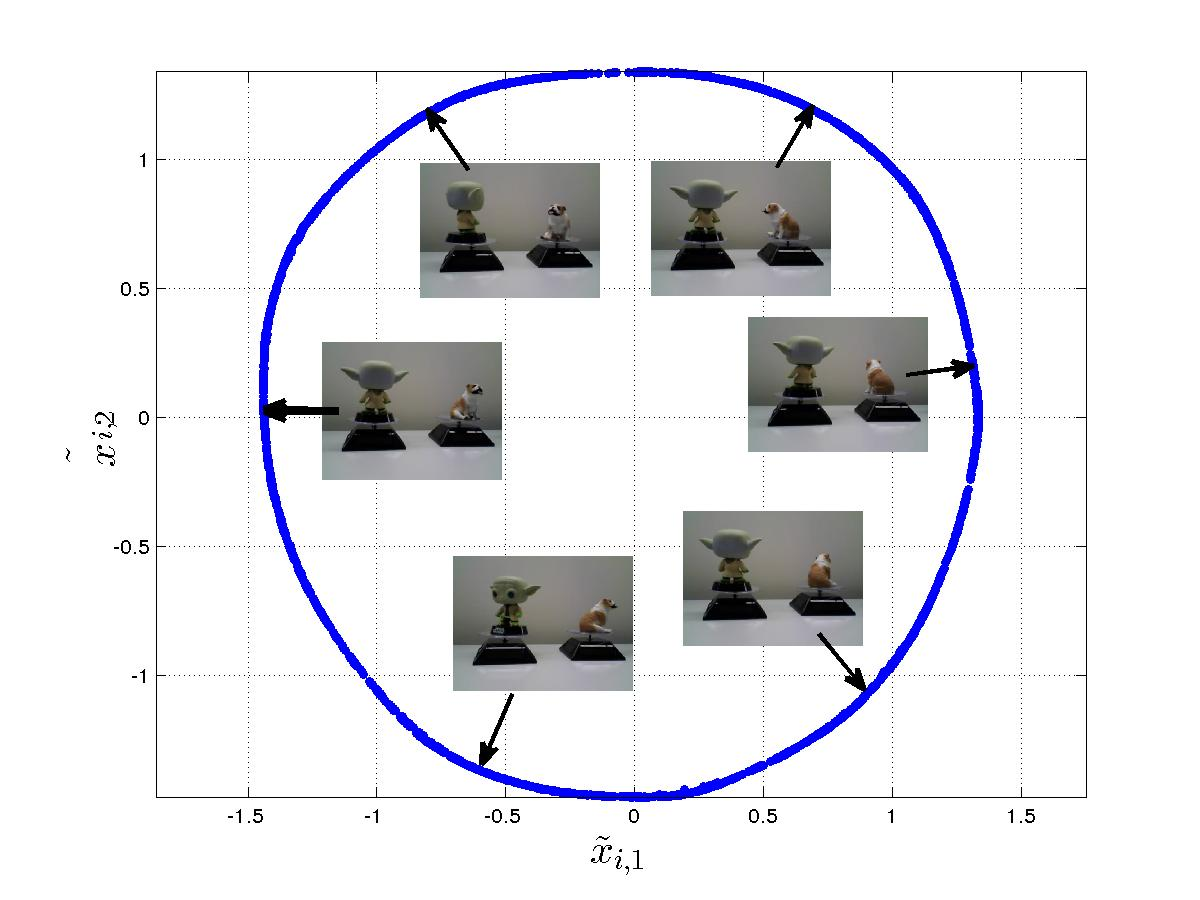# Abstract

One of the challenges in data analysis is to distinguish between different sources of variability manifested in data. In this work, we consider the case of multiple sensors, measuring the same physical phenomenon, so that the properties of the physical phenomenon are manifested as a common source of variability (which we would like to extract) and each sensor has its own sensor-specific effects.
We present a method based on alternating products of diffusion operators, and show that it extracts the common source of variability. Moreover, we show that this method extracts the common source of variability in a multi-sensor experiment as if it were a standard Manifold Learning algorithm used to analyze a simple single-sensor experiment in which the common source of variability is the only source of variability.

A paper describing alternating diffusion:
R.L. and Ronen Talmon. “Learning the geometry of common latent variables using alternating-diffusion.” Applied and Computational Harmonic Analysis (2015).
The technical report: R.L and Ronen Talmon. Common Manifold Learning using Alternating-Diffusion (2014) .

# Demonstration Code and Datasets

Demonstration code and dataset: the spinning figures experiment  [or the larger dataset] (demo code, not optimized for speed or memory). This is a large file with about 8,000 pairs of images (40,000 pairs of images in the larger dataset). The algorithm needs <500 pairs of images, randomly or sparsely chosen from the dataset.

Click to run a small example live (requires a codeocean account):

# Manifold Learning

Manifold Learning methods excel at recovering a low dimensional structure of phenomena from high-dimensional measurements.
To illustrate this, consider the following simple example. We place a puppet on a rotating platform, and take snapshots of the puppet as it rotates (see video in Fig.1). In the absence of prior knowledge about the model, the data we have are images, which are translated to high-dimensional vectors of pixels. However, there is clearly a sense in which the problem is one dimensional; excluding some noise, the only real parameter that sets the images apart is the orientation of the puppet.

Figure 1: one rotating figure, experiment setup.

We used the Manifold Learning technique Diffusion Maps to analyze this dataset of images, the results are presented in Fig. 2 and Fig. 3. Each point in the figure represents one of the snapshots; snapshots depicting the puppet in the same orientation were placed in close proximity by the algorithm, and all the snapshots were arranged in a circle; in this sense, the algorithm captured the one-dimensional nature of dataset.Figure 2: embedding of the data, using diffusion maps.

Figure 3: embedding of the data, using diffusion maps (video).

# Multiple Sensors and the Common Variable Problem

One of the challenges in Manifold Learning is to distinguish between different parameters that are manifested in the data, and in particular, to extract
the parameters that are of interest to the analysis.

In this work, we consider multi-sensor problems, where the same phenomenon is measured by several sensors. Each sensor “sees” the phenomenon, but each also captures some other “irrelevant,” sensor-specific parameters. These “irrelevant” parameters are not restricted to being small additive noise, they can have very strong, non-linear influence on the measurements.

To illustrate the multi-sensor problem, we consider the setup depicted in Fig. 4. and Fig. 5. We have three objects rotating at different speeds, and we have two cameras. Each of the cameras captures two of the objects; both cameras capture the bulldog (from different view angles), but each camera also captures one of the other objects.Figure 4: three rotating objects, experiment setup.

Figure 5: three rotating objects, views from both cameras.

We would use Manifold Learning algorithms to capture all the parameters, because all these parameters are required to describe the manifolds on which the data “lives.” Our goal is to extract the common variable, but this parameter is always mixed with other parameters, and we do not have data “living” on a manifold that can be described by the common variable alone.

We analyzed the set of snapshots taken by Camera 1 using Diffusion Maps, and separately analyzed the snapshots taken by Camera 2 using the same method. Two-dimensional projections of the embedding are presented in Fig. 6. To explain the embedding, we took 4 pairs of snapshots in which the bulldog had the same orientation, and showed where each image was embedded. Both Diffusion Maps are approximately tori (this is easier to see in the case of Camera 1), demonstrating that the Diffusion Maps algorithm capture the two objects seen by each camera.Figure 6: separate Diffusion Maps for the two datasets.

# Alternating Diffusion Example

The method presented here, Alternating Diffusion, extracts the common variable. Since we are trying to extract a representation of a single rotating object, we would expect the representation to be similar to the rotating object in the first experiment, where we only had a single rotating object (Fig. 2). The results from Alternating Diffusion are presented in Fig. 7 and Fig. 8. As demonstrated in the figures, the algorithm extracts the common variable. Now, the images are sorted by the orientation of the bulldog, as if we had an experiment were we had a single camera, looking at a single object.Figure 7: embedding based on Alternating Diffusion. Due to the limited space in the figure, we present only the snapshots taken by Camera 1, however, each point represents a pair of snapshots taken at the same time.

Figure 8: embedding based on Alternating Diffusion, samples sorted by the orientation recovered by Alternating Diffusion.

More information: R.L. and Ronen Talmon. “Learning the geometry of common latent variables using alternating-diffusion.” Applied and Computational Harmonic Analysis (2015).

# A Deep Learning Approach to a Similar Problem

Siamese networks are another way to look at the common variable problem:
Uri Shaham, and R.L. “Common Variable Learning and Invariant Representation Learning using Siamese Neural Networks.” arXiv preprint arXiv:1512.08806 (2015).